## Solving SBT LESSON 3 Triangular vertical prism, quadrilateral standing prism (C3 Math 7 – Horizon) – Math Book

Solving SBT Lesson 3 Triangular vertical prism, quadrilateral standing prism (C3 Math 7 – Horizon)
————

### Solution 1 page 60 SBT Math 7 Creative horizon episode 1 – CTST

Fill in the dot to describe the figure below: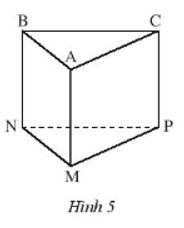– Figure ABC.MNP (Figure 5) is ………….

– The vertices are: ……………………..

– The sides are: …………………….

– The sides are: ……………………………………………………

– The bottom two sides are the ……. and face………….

– The length of side AM is called ……………………. of the prism.

## Detailed instructions for solving Lesson 1

Solution method

Apply properties of sides, angles, vertices, sides of a triangular prism

Detailed explanation

Looking at Figure 5, we can fill in the following:

– Figure ABC.MNP (Figure 5) is figure triangular prism.

– The vertices are: A, B, C, M, N, P.

The side faces are: ABNM, ACPM, BCPN.

– The side edges are: AM, BN, CP.

– The bottom two sides are the sides ABC and face MNP.

– The length of side AM is called height of the prism.

–>

— *****

### Solution 2 page 60 SBT Math 7 Creative horizon episode 1 – CTST

Let the prism stand quadrilateral as Figure 6.

a) Specify the base and side faces of the prism.

b) Name the sides of the prism.

c) The height of the prism is equal to the length of the line segment?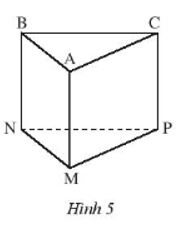## Detailed instructions for solving Lesson 2

Solution method

Using properties of sides and angles of a triangular prism

Detailed explanation

Since the tetragonal vertical prism has rectangular side faces, we have:

a) The two bases of the prism are: the ADHE surface and the BCGF surface. (since these faces are not rectangles, they must be bottom faces).

The four lateral faces of the prism are: surface ABFE, face EFGH, face CDHG and face ABCD. (are rectangles)

b) The sides of the prism are: AB, EF, HG, DC.

c) The height of the prism is equal to the height of the side spacers.

Thus we can say that the height of the prism is equal to the length of line segment AB (or EF, or HG, or DC).

–>

— *****

### Solve problems 3 pages 60 SBT Math 7 Creative horizon episode 1 – CTST

Create a triangular vertical prism with base sides of 2.7 cm, 1.5 cm, 2 cm and a height of 3 cm.

## Detailed instructions for solving Lesson 3

Solution method

– On the card, draw 3 rectangles and 2 triangles with dimensions as shown in Figure 1.

– Fold along the dashed lines we have to create the shape.

Detailed explanation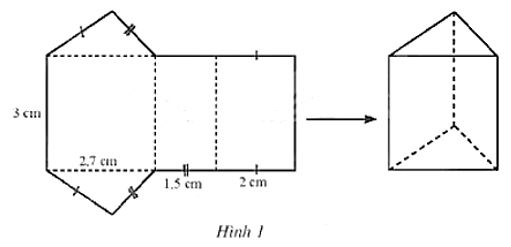–>

— *****

### Solution 4 page 60 SBT Math 7 Creative horizon episode 1 – CTST

Create a quadrilateral vertical prism with measurements as shown in Figure 7.

a) Indicate the height of the prism.

b) Create a prismatic shape.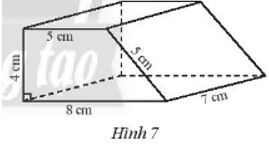## Detailed instructions for solving Lesson 4

Solution method

a) Figure 7 depicts a quadrilateral vertical prism whose side faces are rectangles with dimensions of 5 cm, 7 cm and the base is a square trapezoid with dimensions of 5 cm, 8 cm and 4 cm.

b) On the card, draw 2 trapezoids and 4 rectangles as below, then fold along the dashed lines to get the shape to be created.

Detailed explanation

a) The height of the prism is 7 cm.

b)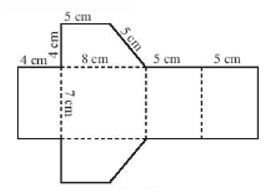–>

— *****

### Solve problem 5 pages 60 SBT Math 7 Creative horizon episode 1 – CTST

Given a triangular prism as shown in Figure 8.

a) What is the height of the prism?

b) Let’s make this triangular prism.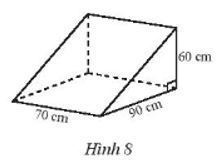## Detailed instructions for solving Lesson 5

Solution method

a) Using properties of the sides of the prism to deduce the height

b) Fold along the dashed line

Detailed explanation

a) A triangular prism has rectangular sides and two bases are triangles.

Therefore, the height of the prism is 70 cm.

b) – On the cardboard draw a rectangle and two right triangles as shown below.

– Fold along the dashed line, we get a triangular prism.

–>

— *****

### Solve lesson 6 pages 60 SBT Math 7 Creative horizon episode 1 – CTST

Show how to cut a rectangular box into two vertical prisms whose base is a trapezoid.

## Detailed instructions for solving Lesson 6

Solution method

Using properties, identifying signs of vertical prism

Detailed explanation

Let ABCD.EFGH rectangular box.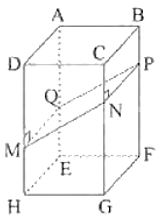– On the segment DH and CG, take two points M, N, so that MN is not perpendicular to CG as shown above.

– Draw a line segment NP perpendicular to CG, and MQ perpendicular to DH.

– Cut along the edges MN, NP, PQ, QM, we get 2 vertical prisms satisfying the requirements.

–>

— *****